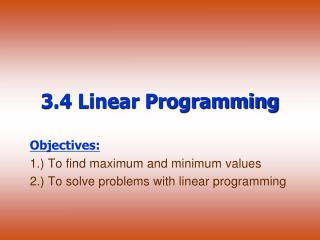DownloadDownload Presentation3.4 Linear Programming

# 3.4 Linear Programming

Télécharger la présentation## 3.4 Linear Programming

- - - - - - - - - - - - - - - - - - - - - - - - - - - E N D - - - - - - - - - - - - - - - - - - - - - - - - - - -
##### Presentation Transcript

1. 3.4 Linear Programming Objectives: 1.) To find maximum and minimum values 2.) To solve problems with linear programming

2. Linear programming got its name because it provides a plan or a program that uses linear equations and inequalities. Vocabulary which means finding the maximum and minimum values of some quantity Many real-life problems involve a process called optimization,___________________________________________________________. In this lesson, we will study one type of optimization process called linearprogramming. A linear programming model consists of a system of linear inequalities called ___________ and an ___________________ that can be can be maximized or minimized. constraints objective quantity

3. Procedure: To find the minimum or maximum value of the objective quantity: • Find all the vertices of the graph of the constraint inequalities. • Evaluate the objective quantity at each vertex. • The least of these is the minimum for the entire region; the greatest of these is the maximum for the region.

4. Example 1Solving a Linear Programming Problem Find the minimum and maximum value of C = 4x + 5y Objective Quantity subject to the following constraints, x ≥ 0 y ≥ 0 Constraints x + y ≤ 6

5. Solution: Step 1:Graph the system of inequalities: x ≥ 0 y ≥ 0 x + y ≤ 6 Step 2:Determine the coordinates of the three vertices of the graph: (__,__)(__,__)(__, __) (0,6) (6,0) (0,0) 0 6 6 0 0 0

6. Solution: Step 3: Evaluate C = 4x + 5y at each of the three vertices of the graph. At (0,0): C = _______________ At (6,0): C = _______________ At (0,6): C = _______________ Step 4: Determine the maximum and minimum values. minimum 0 24 maximum 30

7. Property Vertex Property of Linear Programming Ifthere is a maximum or a minimum value of a linear objective function, it ______________________________________________________________. occurs at one or more vertices of the feasible region.

8. If the region is unbounded, but has a top on it, there will be a maximum only. If the region is unbounded, but has a bottom, there will be a minimum only. A note about: Unbounded Feasible Regions

9. x0 y2x+2 5x+y Vertices? (0,2) C=0+5(2)=10 (1,4) C=1+5(4)=21 Maximum only! Max of 21 at (1,4) Ex: C=x+5y Find the max. & min. subject to the following constraints

10. Check for Understanding Find the minimum value and maximum value of C = 3x + 4y Objective quantity Subject to the following constraints: x ≥ 2 x ≤ 5 Constraints y ≥ 1 y ≤ 6

11. Final Checks for Understanding • Find the maximum and minimum values, if they exist, of c = 3x + 4y for each set of constraints. • 3 ≤ x ≤ 8 b. 2 ≤ x 2 ≤ y ≤ 6 4 ≤ y ≤ 8 2x + y ≥ 12 x + 2y ≥ 16 • Graph the feasible region for each set of constraints: x + 2y ≤ 8 2x + y ≥ 10 x ≥ 0 y ≥ 0 No max min = 34 max = 48 min = 23

12. Real-Life Application: Manufacturing A ski manufacturer, which has a fabricating and a finishing department, makes two types of skis. A pair of downhill skis requires 6 hours to fabricate and 1 hour to finish. A pair of cross-country skis requires 4 hours to fabricate and 1 hour to finish. The fabricating department has 108 hours of labor available per day, while the finishing department has 24 hours of labor available per day. The company makes a profit of \$40 on each pair of downhill skis and \$30 profit on each pair of cross-country skis.

13. Write the objective function. Write a system of linear inequalities to represent the constraints. Graph the feasible region. Find the maximum profit for the given constraints. Real-Life Application: Manufacturing

14. Solution: Let x = downhill skis Let y = cross-country skis 6x + 4y ≤ 108 x + y ≤ 24 x ≥ 0 y ≥ 0 P(rofit) = 40x + 30 y; Maximum _________ \$780